Question

Electric Field Due to Two Charges what is the the electric field at a point midway...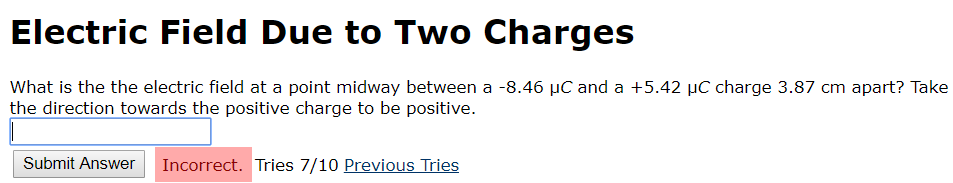Electric Field Due to Two Charges what is the the electric field at a point midway between a-8.46 μC and a +5.42 μC charge 3.87 cm apart? Take the direction towards the positive charge to be positive. Submit Answer Incorrect. Tries 7/10 Previous Tries

Consider two opposite charges placed at a distance d, here I assumed the negative charge on the left side and the positive on the right side.

Given that, q1 = -8.6 x 10-6 C, q2 = +5.42 x 10-6 C and distance of separation d = 3.87 cm = 0.0387 m and d/2 = 0.01935

The electric file due to the charge q1 at a point midway between them is E1 = k (-8.6 x 10-6 C)./ (0.01935 m)2 = -2.06 x 108 N/C and its direction is pointing left because E fields point in towards negative charges. That means towards left side.

since k = 9 x 109 N m2/C2

and the electric field due to the charge q2 at the mid point is E2 = k (+5.42 x 10-6 C) / (0.01935 m)2 = +1.30 x 108 N/C and its direction pointing towards left because E fields point away from positive charges. That means E2 towards left side.

Therefore, the net electric field at the mid point is Enet = E1 + E2.

From the given question, we need to consider that the direction towards the positive charge to be positive. That means the direction towards the negative charge will be negative. So the right side will be negative.

Using the sign convention given in the question, the net field's direction pointing towards right side, that means Enet has an negative sign.

Therefore, Enet = E1 + E2 = -2.06 x 108 N/C + 1.30 x 108 N/C = 0.76 x 108 N/C and its direction is pointing towards left or towards negative charge, so Enet should have negative sign. Therefore, Enet = -0.76 x 108 N/C.

Earn Coins

Coins can be redeemed for fabulous gifts.

Similar Homework Help Questions
• Part A What is the magnitude of the electric field at a point midway between a-5.6...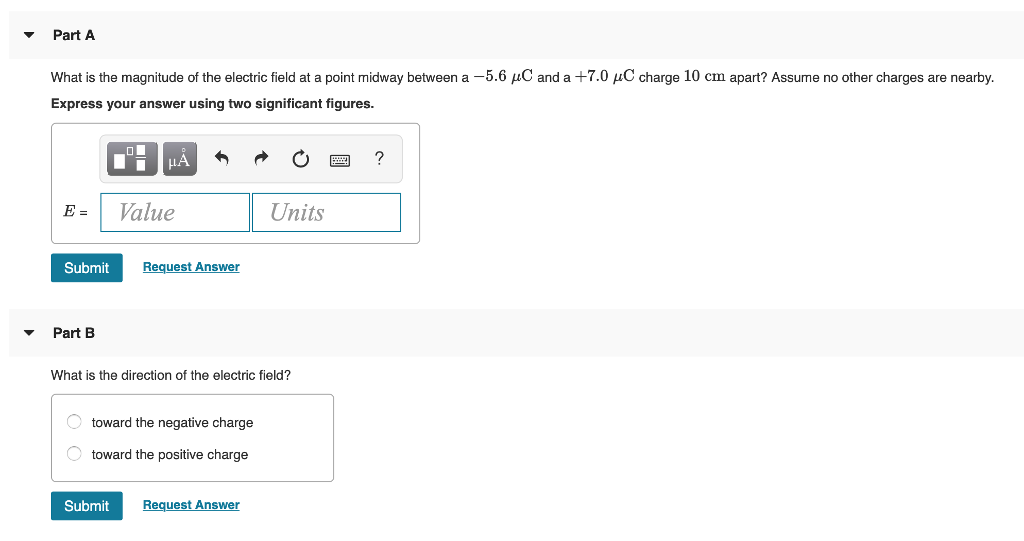Part A What is the magnitude of the electric field at a point midway between a-5.6 μC and a +7.0 μC charge 10 cm apart? Assume no other charges are nearby Express your answer using two significant figures. B-1 Value Units Submit Request Answer Part B What is the direction of the electric field? toward the negative charge toward the positive charge Submit Request Answer

• Electric Field a the Mid-Point of Two Charges The electric field midway between two equal but...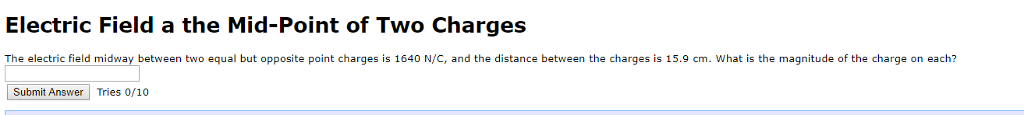Electric Field a the Mid-Point of Two Charges The electric field midway between two equal but opposite point charges is 1640 N/C, and the distance between the charges is 15.9 cm. What is the magnitude of the charge on each? Submit Answer Tries 0/10

• What is the magnitude of the electric field at a point midway between a-7.4 μC and...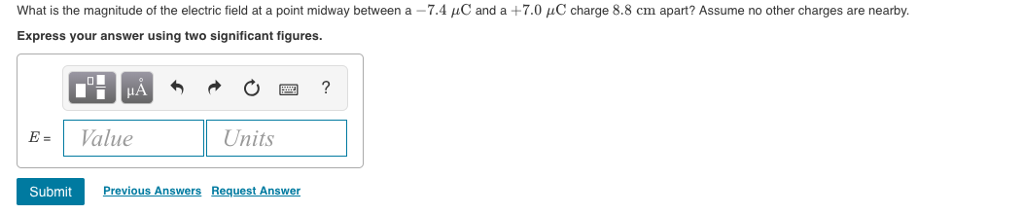What is the magnitude of the electric field at a point midway between a-7.4 μC and a +7.0 μC charge 8.8 cm apart? Assume no other charges are nearby. Express your answer using two significant figures 이-? = | Value Units Submit

• What is the magnitude of the electric field at a point midway between a −6.8μC and...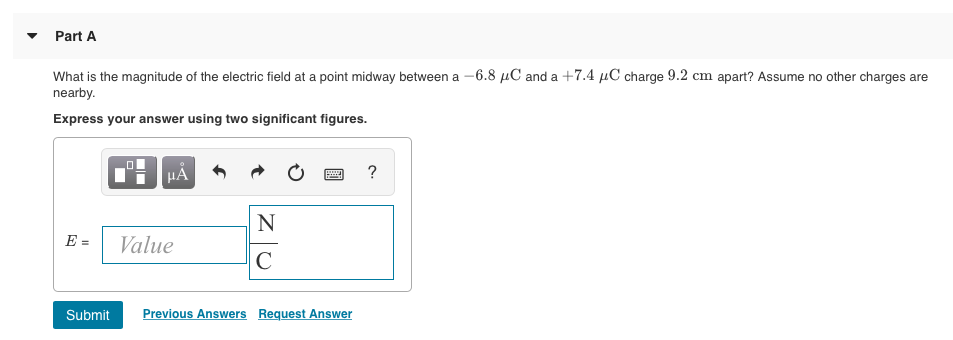What is the magnitude of the electric field at a point midway between a −6.8μC and a +7.4μC charge 9.2cm apart? Assume no other charges are nearby. Part A What is the magnitude of the electric field at a point midway between a -6.8 C and a +7.4 HC charge 9.2 cm apart? Assume no other charges are nearby. Express your answer using two significant figures. E- 1 alue Previous Answers Request Answer

• What is the magnitude of the electric field at a point midway between a -7.7 uC...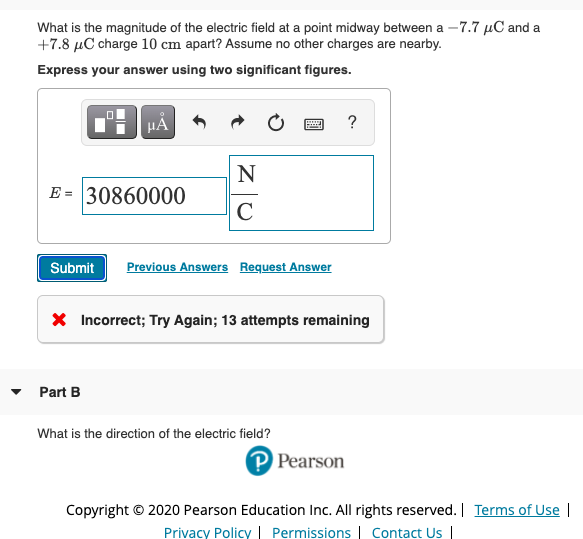What is the magnitude of the electric field at a point midway between a -7.7 uC and a +7.8 C charge 10 cm apart? Assume no other charges are nearby. Express your answer using two significant figures. IP μΑ ? N E = 30860000 С Submit Previous Answers Request Answer X Incorrect; Try Again; 13 attempts remaining Part B What is the direction of the electric field? Pearson Copyright © 2020 Pearson Education Inc. All rights reserved. Terms of Use...

• What is the magnitude of the electric field at a point midway between a 6.2 μ...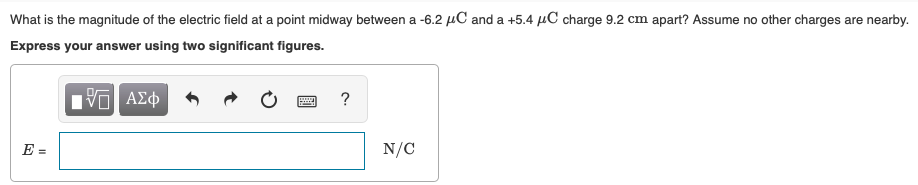What is the magnitude of the electric field at a point midway between a 6.2 μ and a +5.4 μC charge 9.2 cm apart? Assume no other charges are nearby Express your answer using two significant figures. N/C

• The figure shows two point charges. Calculate the magnitude of the electric field at point P....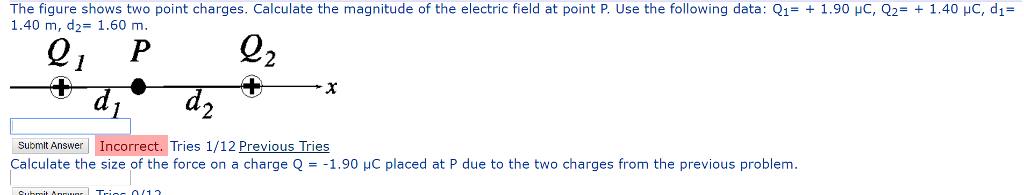The figure shows two point charges. Calculate the magnitude of the electric field at point P. Use the following data: Q1-+ 1.90 μC, Q2-+ 1.40 μ。 , d,- 1.40 m, d2-1.60 m. submit Answer Incorrect. Tries 1/12 Previous Tries Calculate the size of the force on a charge Q--1 .90 μC placed at P due to the two charges from the previous problem.

• ​What is the magnitude of the electric field at a point midway between a -6.5 μC and a +5.0 μC charge 8.4 cm apart?

What is the magnitude of the electric field at a point midway between a -6.5 μC and a +5.0 μC charge 8.4 cm apart? Assume no other charges are nearby. Express your answer using two significant figures. What is the direction of the electric field at the corner? along the line between the corner and the center of the square toward the center along the side of the square between the corner and one of the charges toward the charge along the side of the...

• Part A What is the magnitude of the electric field at a point midway between a...

Part A What is the magnitude of the electric field at a point midway between a −8.5μC and a +5.0μC charge 10cm apart? Assume no other charges are nearby. Express your answer using two significant figures. E= Part B What is the direction of the electric field? a. toward the positive charge b. toward the negative charge

• What is the magnitude of the electric field at a point midway between a −8.0μC and...

What is the magnitude of the electric field at a point midway between a −8.0μC and a +7.8μC charge 9.8cm apart? Assume no other charges are nearby. Is the direction of the electric field to the negative or positive charge?